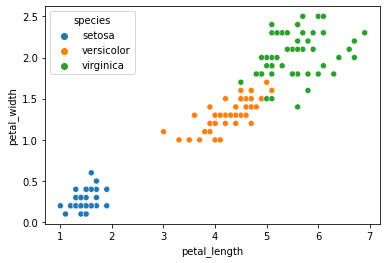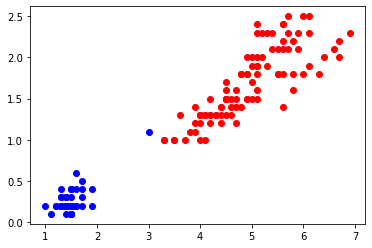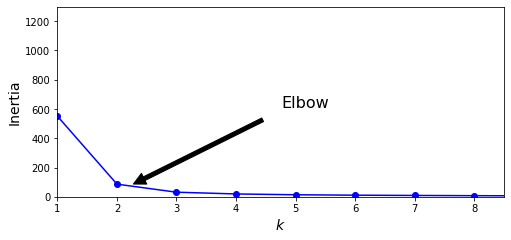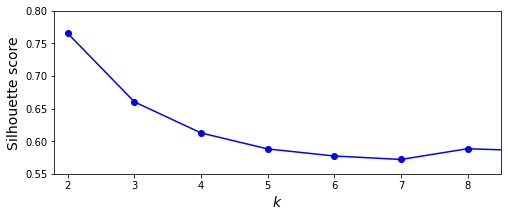# 机器学习(六)：通俗易懂无监督学习K-Means聚类算法及代码实践

K-Means是一种无监督学习方法，用于将无标签的数据集进行聚类。其中K指集群的数量，Means表示寻找集群中心点的手段。

# 一、 无监督学习 K-Means

1. 随便选择K个中心点（大哥）。
2. 把距离它足够近的数据（小弟）吸纳为成员，聚成K个集群（组织）。
3. 各集群（组织）内部重新选择中心点（大哥），选择标准是按照距离取均值作为中心点（大哥）。
4. 重复2、3步骤直到收敛（组织成员相对稳定）。

# 二、 K-Means代码实践

## 2.1 鸢尾花数据集

import numpy as np
import pandas as pd
import seaborn as sns
import matplotlib.pyplot as plt

# 大家不用在意这个域名
sns.scatterplot(x='petal_length',y='petal_width',data=df,hue='species')## 2.2 K-Means训练数据

X = df[['petal_length','petal_width']].to_numpy()

from sklearn.cluster import KMeans
k = 2
kmeans = KMeans(n_clusters=k, random_state=42)
y_pred = kmeans.fit_predict(X)
plt.plot(X[y_pred==1, 0], X[y_pred==1, 1], "ro", label="group 1")
plt.plot(X[y_pred==0, 0], X[y_pred==0, 1], "bo", label="group 0")
# plt.legend(loc=2)
plt.show()# 三、K的选择

## 3.1 惯性指标（inertia）

K-Means的惯性计算方式是，每个样本与最接近的集群中心点的均方距离的总和

kmeans_per_k = [KMeans(n_clusters=k, random_state=42).fit(X)
for k in range(1, 10)]

inertias = [model.inertia_ for model in kmeans_per_k]
plt.figure(figsize=(8, 3.5))
plt.plot(range(1, 10), inertias, "bo-")
plt.xlabel("$k$", fontsize=14)
plt.ylabel("Inertia", fontsize=14)
plt.annotate('Elbow',
xy=(2, inertias),
xytext=(0.55, 0.55),
textcoords='figure fraction',
fontsize=16,
arrowprops=dict(facecolor='black', shrink=0.1)
)
plt.axis([1, 8.5, 0, 1300])
plt.show()## 3.2 轮廓系数指标（silhouette）

K-Means的轮廓系数计算方式是，与集群内其他样本的平均距离记为a，与外部集群样本的平均距离记为b，轮廓系数(b-a)/max(a,b)

from sklearn.metrics import silhouette_score
silhouette_scores = [silhouette_score(X, model.labels_)
for model in kmeans_per_k[1:]]
plt.figure(figsize=(8, 3))
plt.plot(range(2, 10), silhouette_scores, "bo-")
plt.xlabel("$k$", fontsize=14)
plt.ylabel("Silhouette score", fontsize=14)
plt.axis([1.8, 8.5, 0.55, 0.8])
plt.show()# 四、自动选择K值（拓展）

## 4.1 简单理解贝叶斯定理

$P(A|B) = \frac{P(A)P(B|A)}{P(B)}$
 其中，P(A) 表示事件A发生的概率，P(A B)表示事件B发生时事件A发生的概率。上面的公式中B就是所谓的证据。这里要注意的是，P(B)的出现让P(A B)变的更确定了，并不是说概率变高了或者变低了。概率的高或者低都是一种确定。它是一种信心程度的体现。

## 4.2 贝叶斯高斯混合模型

from sklearn.mixture import BayesianGaussianMixture
bgm = BayesianGaussianMixture(n_components=10, n_init=10, random_state=42)
y_pred = bgm.fit_predict(X)
np.round(bgm.weights_, 2)